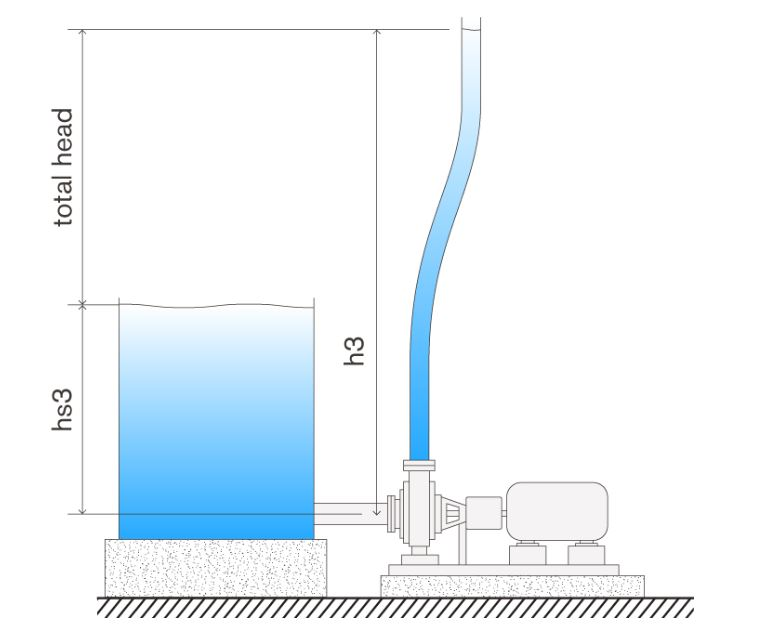Pumps, How they Work? And What is the Head?Source: New York Engineers

When it comes to pumps, one of the most misunderstood terms is that of the pump head. In several professional interviews, this is always an often posed topic.Source: amarineblog

The cumulative mechanical energy along a streamline is always constant, according to Bernoulli's theorem. The number of the pressure head, kinetic head, and potential head is the overall mechanical energy.

For a fluid to move, the total mechanical energy at point 1 must be greater than the total mechanical energy at point 2.

The height at which a pump can send fluid (only liquid) in a vertical direction versus gravity is referred to as the pump's head. The height and everything else are calculated by the pump's efficiency. If a pump can generate more pressure, it can send the fluid to a greater height.Source: globalpumps

Consider the following diagram, which shows a pump attached to a sump and a vertical tube in the discharge. Simply put, the head of a pump is the highest height that it can reach while pumping against gravity.

The concern now is whether the suction conditions impact the head.Source: globalpumps

Yes, that is the answer. The head value reduces as the suction level is reduced. The reverse is also true: as the suction level is raised, the head value grows.

In all cases, the total head (difference between discharge and suction head) remains unchanged.

The head height is measured using the assumption that the impeller's eye height is zero.

The pump's motor essentially transforms electric energy into mechanical energy, which is then used by the pump to spin the impeller mounted on the motor's shaft, which then transfers the mechanical energy to the fluid of interest. Thanks to the geometrical structure of the pump known as the volute, the fluid now has kinetic energy that is being transformed into pressure energy.

As we know, pressure equals the density of the fluid multiplied by the acceleration due to gravity multiplied by the height.

As a consequence, pressure is proportional to height.

We change the potential energy of the fluid by raising or lowering the suction level of the fluid. The potential energy of a ten-foot water column is higher than the potential energy of a five-foot water column. And this potential head aids the impeller in imparting more mechanical energy, resulting in more head.

As a result, increasing the amount of liquid in the suction tank will result in a higher head, while lowering the level will result in a lower head.

Another feature of the head is that it is unaffected by the form of fluid being pumped (assuming the viscosity is relatively low and similar to water). The head reached would be the same if you're pumping water or a heavy caustic solution. The heavier solution, on the other hand, would have a greater pressure at the pump's discharge.

When it comes to choosing a pump, there are two things to consider:

1. The overall head
2. What is the flow rate that you require?

### Head Shut Off :

It is known as the pump's maximum head when the discharge is held at zero.

Shut off head can be determined using the following formula:

As we all know, when fluid flows into a pipe, the pressure reaches the system, stopping the fluid from achieving its optimal flow rate.

The amount of total head that the pump can achieve is reduced as a result of the friction. In fact, as the flow increases, so does friction, and the overall head decreases. The amount of head lost as a result of friction is referred to as "friction head" or "friction-loss."

The overall head of a running system is the difference between the discharge and suction heads plus the friction head, which is smaller than the shut-off head.

It's important to understand that friction loss is proportional to the length and diameter of the pipe in which the fluid flows. If the loss is significant, it can be calculated using the Darcy Weisbatch equation.